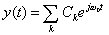# Fourier Series

Up until now, we have only looked at signals in the time (or space) domain.

We will now transform signals to the frequency domain. The reason for doing this is that many signals are more convenient to process, analyze, synthesize, and/or compress in the frequency domain.

Here are some examples of common signal processing operations that you may be familiar with:

Real-Life Example 1 Image Compression

It is well known that the human eye is less sensitive to errors in high spatial frequencies. As a result, we can obtain good image quality at low bit rates using the discrete cosine transform (DCT) by closely reproducing the low frequencies of the image while saving bits (and thus allowing more error) in the high frequencies. The DCT is used in the JPEG and MPEG image and video compression standards. It is also used in RealNetworks' video codecs. (It is related to the discrete Fourier transform (which you may study in later courses) except the exponential in the kernel is replaced by a cosine when taking the transform.)

Real-Life Example 2 Speech Recognition.

By displaying a speech spectrogram (a plot of intensity of frequency vs. time), researchers at MIT can determine what speech utterance was used to produce the spectrogram. This is a crude way of performing speech recognition.

Real-Life Example 3 Samping a continuous time signal.

By knowing the highest frequency in a signal, we can determine the rate at which to sample the analog signal to ensure that the original signal can be recovered from the digitized samples. We will see when we discuss the sampling theorem that we must sample at least twice the highest frequency in the signal to avoid aliasing. Along these lines, music is sampled at 44.1 kHz when it is recorded on a CD, because the human ear can only hear frequencies up to about 20 kHz.

## Approximating Periodic Functions

During our study of the Fourier Series, we will see how to build and approximate periodic functions by a (possibly infinite) sum of sinusoidal signals.

### Periodic Functions

If x(t) = x(t+T), then x(t) is periodic with period T.

For example, the signal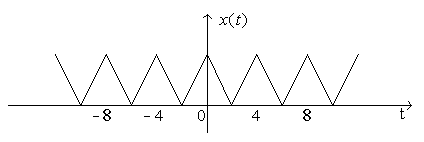is periodic with T=4.

We will see how to approximate periodic signals with complex exponentials.

## Fourier Series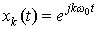are harmonic signals with period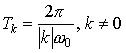Fourier Series idea: We can represent all periodic signals as a harmonic series of the form: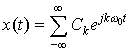where the Ck are the Fourier Series coefficients and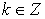k = 0 gives the DC (zero frequency) signal

k ± 1 yields the fundamental frequency, which is also known as the first harmonic ω0

|k|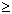2 are harmonics

There are a number of different forms a Fourier Series can take. They are equivalent. Here, we will work with the Exponential form.

Example 1 Find the Fourier Series coefficients for

x(t) = cos(ω0t) + sin(2ω0t)

Example 2 Find the Fourier Series coefficients for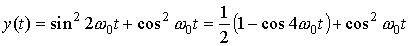Example 3 SYNTHESIS

 Given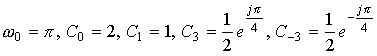, find the signal